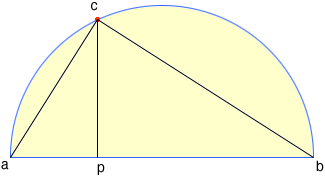SEARCH HOMEMath Central Quandaries & QueriesQuestion from arun, a student: a semi circle is drawn with ab as diameter from p a point on ab a line perpendicular to ab is drawn meeting circumference of semi circle at c, ac = 2cm, cd = 6cm find are of the semi circle?Hi Arun,

I drew a diagram of the situation you described.Since the line segment ab is a diagonal of a circle and c is on the circumference, the angle bca is a right angle. You know the lengths of bc and ca so you can use Pythagoras theorem to find the length of the diagonal ab.

PennyMath Central is supported by the University of Regina and The Pacific Institute for the Mathematical Sciences.